# AP Board 7th Class Maths Solutions Chapter 8 Exponents and Powers InText Questions

SCERT AP 7th Class Maths Solutions Pdf Chapter 78 Exponents and Powers InText Questions and Answers.

## AP State Syllabus 7th Class Maths Solutions 8th Lesson Exponents and Powers InText Questions

Check Your Progress [Page No. 28]

Question 1.
Write the following in exponential form by using 10 as the base number :
(i) 10,00,00,000
(ii) 100,00,00,000

 Number Expanded form Exponential (i) 10,00,00,000 10 × 10 × 10 × 10 × 10 × 10 × 10 × 10 108 (ii) 100,00,00,000 10 × 10 × 10 × 10 × 10 × 10 × 10 × 10 × 10 109

Let’s Explore [Page No. 29]

Question 1.
Observe and complete the following table. First one is done for you.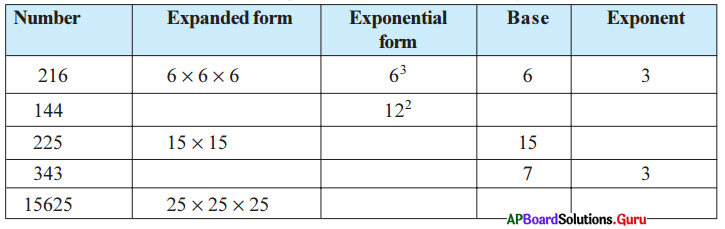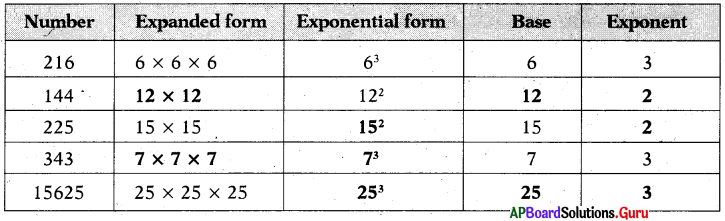Question 2.
Write the following numbers in exponential form. Also state the base, exponent and how to read. .
(i) 16
(ii) 49
(iii) 512
(iv) 243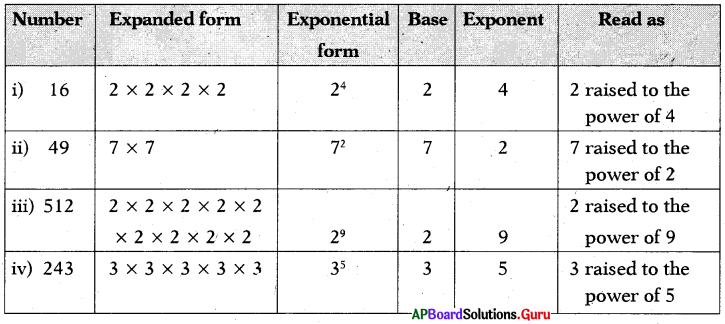Question 3.
Compute the following and write the greater one.
(i) 43 or 34
43 = 4 × 4 × 4 = 64
34 = 3 × 3 × 3 × 3 = 81 is greater.
64 < 81 (or) 81 > 64
So, 43 < 34 (or) 34 > 43

(ii) 53 or 35
53 = 5 × 5 × 5 = 125
35 = 3 × 3 × 3 × 3 × 3 = 243 is greater.
125 < 243 (or) 243 > 125
So, 53 < 35 (or) 35 > 53Question 4.
32 = 3 × 3 = 9
23 = 2 × 2 × 2 = 8
9 ≠ 8
32 ≠ 23
So, ab ≠ ba unless a = b

Check Your Progress [Page No. 30]

Express the following number in exponential form using prime factorisation:
(i) 432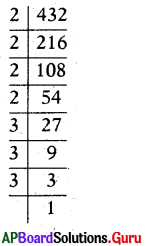432 = 2 × 216
= 2 × 2 × 108
= 2 × 2 × 2 × 54
= 2 × 2 × 2 × 2 × 27
= 2 × 2 × 2 × 2 × 3 × 9
= 2 × 2 × 2 × 2 × 3 × 3 × 3
∴ 432 = 24 × 33

(ii) 1296
1296 = 2 × 648
= 2 × 2 × 324
= 2 × 2 × 2 × 162
= 2 × 2 × 2 × 2 × 81
= 2 × 2 × 2 × 2 × 3 × 27
= 2 × 2 × 2 × 2 × 3 × 3 × 9
= 2 × 2 × 2 × 2 × 3 × 3 × 3 × 3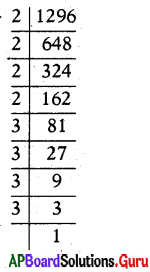∴ 1296 = 24 × 34

(iii) 729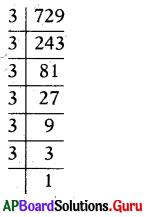729 = 3 × 243
= 3 × 3 × 81
= 3 × 3 × 3 × 27
= 3 × 3 × 3 × 3 × 9
= 3 × 3 × 3 × 3 × 3 × 3
729 = 36

(iv) 1600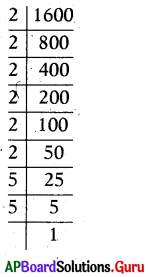1600 = 2 × 800
= 2 × 2 × 400
= 2 × 2 × 2 × 200
= 2 × 2 × 2 × 2 × 100
= 2 × 2 × 2 × 2 × 2 × 50
= 2 × 2 × 2 × 2 × 2 × 2 × 25
= 2 × 2 × 2 × 2 × 2 × 2 × 5 × 5
1600 = 26 × 52

Let’s Explore [Page No. 32]

Question 1.
Write the appropriate number in place of ▢ in the following.
Let ‘b’ be any non-zero integer.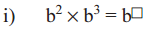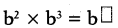= b2 × b3
= b × b × b × b × b = b5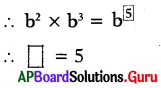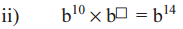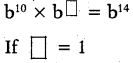then b10 × b1 = b14
b10+1 = b14
b11 ≠ b14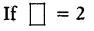then b10 × b2 = b14
b10+2 = b14
b12 ≠ b14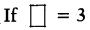then b10 × b3 = b14
b10+3 = b14
b13 ≠ b14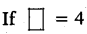then b10 × b4 = b14
b10+4 = b14
∴ b14 = b14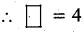Question 2.
Simplify the following using the formula am × an = am+n
(i) 57 × 54
57 × 54
We know that am × an = am+n;
57 × 54 = 57+4 = 511
∴ 57 × 54 = 511

(ii) p3 × p2
p3 × p2
We know that am × an = am+n
p3 × p2 = p3+2 = p5
∴ p3 × p2 = p5

(iii) (-4)10 × (-4)3 × (-4)2
(-4)10 × (-4)3 × (-4)2
We know that am × an = am+n
(- 4)10+3+2 = (- 4)15
∴ (-4)10 × (-4)3 × (-4)2 = (- 4)15

Let’s Explore [Page No. 33]

Question 1.
Write the following in exponential form using the formula (am)n = amn.
(i) (62)4
(62)4
We know (am)n = amn
(62)4 = 62×4 = 68
∴ (62)4 = 68

(ii) (22)100
(22)100
We know (am)n = amn
(22)100 = 22×100 = 2200
∴ (22)100 = 2200

(iii) (206)2
(206)2
We know (am)n = amn
(206)2 = 206×2 = 2012
∴ (206)2 = 2012

(iv) [(-10)3]5
[(-10)3]5
We know (am)n = amn
[(-10)3]5 =(-10)3×5 = (-10)15
∴ [(- 10)3]5 = (- 10)15Check Your Progress [Page No. 34]

Simplify the following by using the law , am × bm = (ab)m.
(i) 76 × 36
76 × 36
We know, am × bm = (ab)m
76 × 36 = (7 × 3)6 = (21)6
∴ 76 × 36 = 216

(ii) (3 × 5)4
(3 × 5)4
We know, (ab)m = am × bm
(3 × 5)4 = 34 × 54
∴ (3 × 5)4 = 34 × 54

(iii) a4 × b4
a4 × b4
We know, am × bm = (ab)m
a4 × b4 = (a . b)4
∴ a4 × b4 = (a . b)4

(iv) 32 × a2
32 × a2
We know, am × bm = (ab)m
32 × a2 = (3 × a)2 = (3a)2
∴ 32 × a2 = (3a)2

Let’s Explore [Page No. 37]

Question 1.
Simplify and write In the form of am-n Or $$\frac{1}{\mathbf{a}^{\mathbf{n}-\mathbf{m}}}$$
(i) $$\frac{10^{8}}{10^{4}}$$
We Know $$\frac{10^{8}}{10^{4}}$$ = am-n
$$\frac{10^{8}}{10^{4}}$$ = 108-4 = 104
∴$$\frac{10^{8}}{10^{4}}$$ = 104

(ii) $$\frac{(-7)^{13}}{(-7)^{10}}$$
$$\frac{(-7)^{13}}{(-7)^{10}}$$
We Know $$\frac{a^{m}}{a^{n}}$$ = am-n (m > n)
$$\frac{(-7)^{13}}{(-7)^{10}}$$ = (-7)13-10
= (-7)3 = -7 × -7 × -7 = – 343
∴$$\frac{(-7)^{13}}{(-7)^{10}}$$ = – 343

(iii) $$\frac{12^{5}}{12^{8}}$$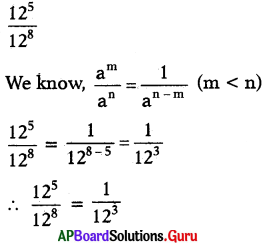(iv) $$\frac{3^{4}}{3^{7}}$$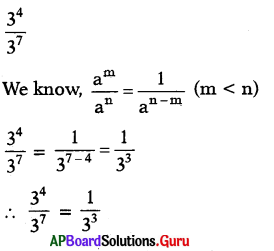Question 2.
Fill the appropriate number In the box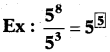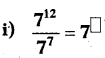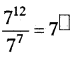We Know $$\frac{a^{m}}{a^{n}}$$ = am-n (m > n)
$$\frac{7^{12}}{7^{7}}$$ = 712-7 = 75
∴ $$\frac{7^{12}}{7^{7}}$$ = 75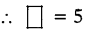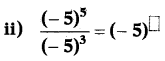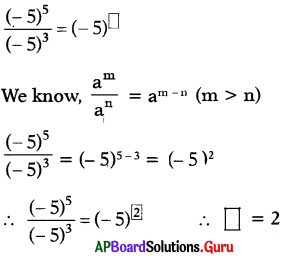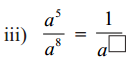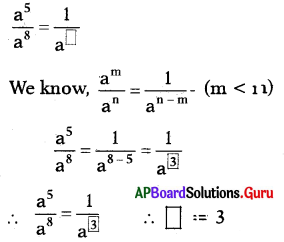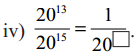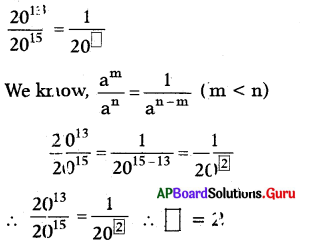Question 3.
Simplify the following :
(i) $$\frac{6^{8}}{6^{8}}$$
$$\frac{6^{8}}{6^{8}}$$
We Know $$\frac{a^{m}}{a^{n}}$$ = am-n
$$\frac{6^{8}}{6^{8}}$$ = 68-8 = 60 = 1 (∵ a0 = 1)

(ii) $$\frac{t^{10}}{t^{10}}$$
$$\frac{t^{10}}{t^{10}}$$
We Know $$\frac{a^{m}}{a^{n}}$$ = am-n
$$\frac{t^{10}}{t^{10}}$$ = t10-10 = t0 = 1 (∵ a0 = 1)

(iii) $$\frac{12^{7}}{12^{7}}$$
$$\frac{12^{7}}{12^{7}}$$
We Know $$\frac{a^{m}}{a^{n}}$$ = am-n
$$\frac{12^{7}}{12^{7}}$$ = 127-7 = 120 = 1 (∵ a0 = 1)

(iv) $$\frac{p^{5}}{p^{5}}$$
$$\frac{p^{5}}{p^{5}}$$
We Know $$\frac{a^{m}}{a^{n}}$$ = am-n
$$\frac{p^{5}}{p^{5}}$$ = p10-10 = p0 = 1 (∵ a0 = 1)

Check Your Progress [Page No. 38]

Question 1.
Complete the following boxes .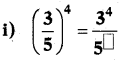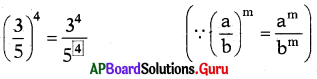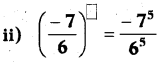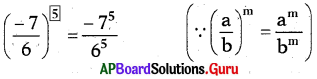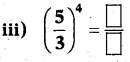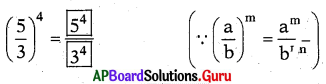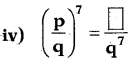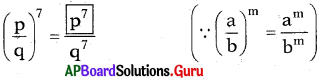Check Your Progress [Page No. 39]

Question 1.
Express the following in exponential form.
(i) $$\frac{-27}{125}$$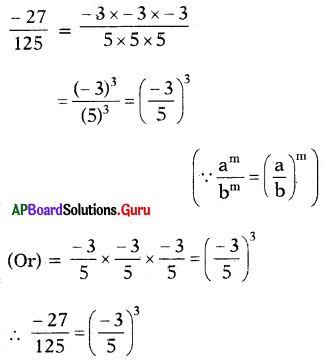(ii) $$\frac{-32}{243}$$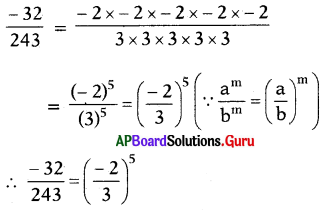(iii) $$\frac{-125}{1000}$$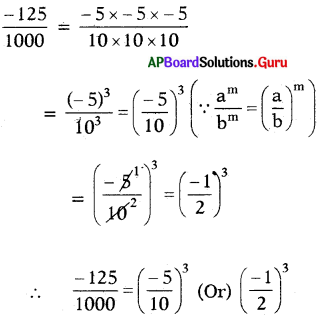(iv) $$\frac{-1}{625}$$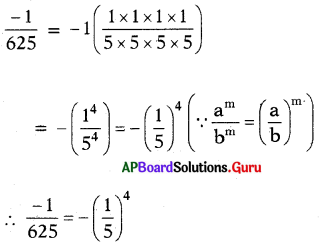Let’s Think [Page No. 39]

Question 1.
Deekshltha and Harsha computed 4(3)2 in different ways.
Deeksbitha did it like this
4(3)2 = (4 × 3)2
= 122
= 144

Harsha did it like this
4(3)2 = 4 × (3 × 3)
= 4 × 9
= 36
Who has done the problem Incorrectly?
Discuss the reason for the mistake with your friends.
In 4(3)2 square only belongs to 3, but not 4.
So, Deekshitha did wrong and Harsha did correct.

Let’s Do Activity [Page No. 40]

Finding the pair : Divide the classroom into two groups. Each group has a set of cards. Each student of group 1 has to pair with one suitable student of group 2 by stating in the reason.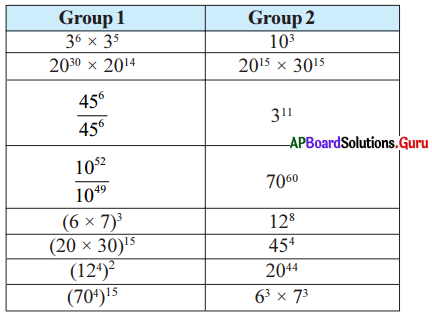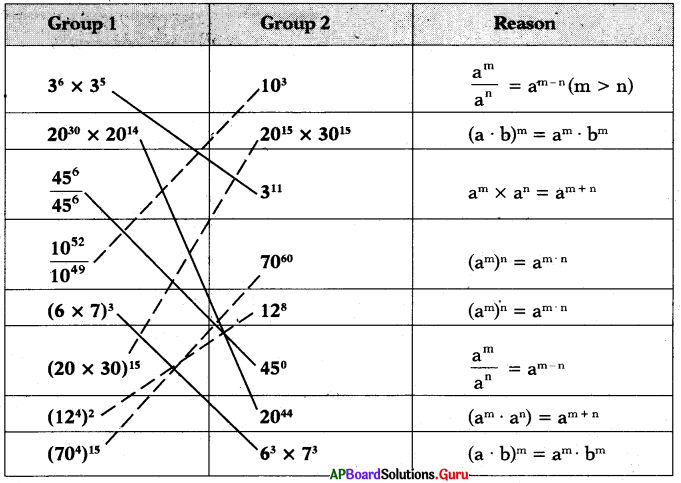Note : This activity can be extended till all the children in the class are familiarised with the laws of exponents.

Project Work [Page No. 42]

Collect the annual income of 5 families in your location by observing their ration card and rounded into the nearest thousand / Lakh and express in the exponential form. One done for you.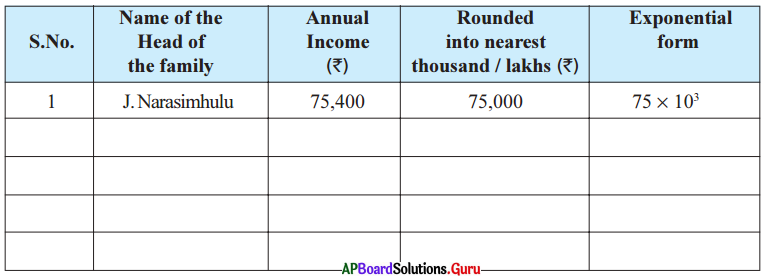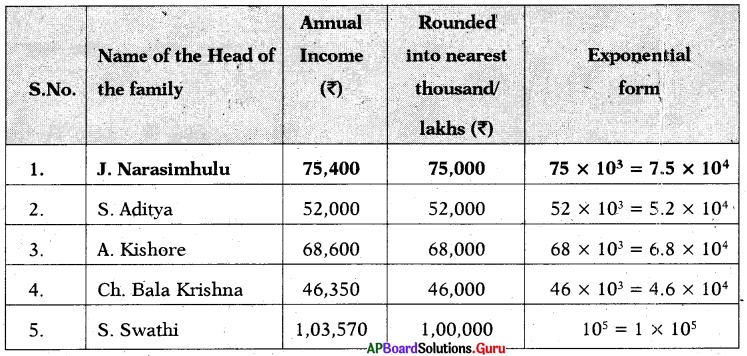Examples:

Question 1.
Which one is greater 82 or 28? Justify.
82 = 8 × 8 = 64
28 = 2 × 2 × 2 × 2 × 2 × 2 × 2 × 2 = 256
256 >64
Therefore, 28 > 82.

Question 2.
Simplify the following using the formula am × an = am+n
(i) (- 5)7 × (- 5)4
(- 5)7 × (- 5)4 = (- 5)7+4
(∵ am × an = am+n)
= (- 5)11
∴ (- 5)7 × (- 5)4 = (- 5)11

(ii) 33 × 32 × 34
33 × 32 × 34 = 33+2+4
(∵ am × an = am+n)
= 39
∴ 33 × 32 × 34 = 39Question 3.
Simplify the following using the formula (am)n = amn
(i) (83)4
(83)4 = 83×4
= 812
∴ (83)4 = 812

(ii) [(-11)5]2
[(-11)5]2 = (- 11)5×2
= (- 11)10
∴ [(-11)5]2 = (- 11)10

(iii) (750)2
(750)2= 750×2 = 7100
∴ (750)2 = 7100

Question 4.
Simplify the following using the expo-nential law am × bm = (ab)m
(i) 52 × 32
52 × 32 = (5 × 3)2 [∵ am × bm = (ab)m]

(ii) p3 × q3
p3 × q3 = (p × q)3

(iii) (7 × 8)4
(7 × 8)4 = 74 × 84 [∵ (ab)m = am × bm]

Question 5.
Simplify the following and write in the form of $$\frac{a^{m}}{a^{n}}$$ = am-n or $$\frac{a^{m}}{a^{n}}=\frac{1}{a^{n-m}}$$
(i) $$\frac{2^{9}}{2^{3}}$$
$$\frac{2^{9}}{2^{3}}$$ = 29-3 [∵ $$\frac{a^{m}}{a^{n}}$$ = am-n]
= 26

(ii) $$\frac{(-9)^{11}}{(-9)^{7}}$$
$$\frac{(-9)^{11}}{(-9)^{7}}$$ = (- 9)11-7 = (-9)4

(iii) $$\frac{7^{10}}{7^{13}}$$
$$\frac{7^{10}}{7^{13}}=\frac{1}{7^{13-10}}$$
[∵ $$\frac{a^{m}}{a^{n}}=\frac{1}{a^{n-m}}$$]
= $$\frac{1}{7^{3}}$$

(iv) $$\frac{6^{2}}{6^{5}}$$
$$\frac{6^{2}}{6^{5}}=\frac{1}{6^{5-2}}=\frac{1}{6^{3}}$$

Question 6.
Simplify the following by using formula $$\frac{a^{m}}{b^{m}}=\left(\frac{a}{b}\right)^{m}$$.
(i) $$\frac{5^{3}}{2^{3}}$$
$$\frac{5^{3}}{2^{3}}=\left(\frac{5}{2}\right)^{3}$$ [∵ $$\frac{a^{m}}{b^{m}}=\left(\frac{a}{b}\right)^{m}$$]

(ii) $$\left(\frac{8}{5}\right)^{4}$$
$$\left(\frac{8}{5}\right)^{4}=\frac{8^{4}}{5^{4}}$$
[∵ $$\frac{a^{m}}{b^{m}}=\left(\frac{a}{b}\right)^{m}$$]

Question 7.
Evaluate:
(1)4, (1)5, (1)7, (- 1)2, (- 1)3, (- 1)4, (- 1)5
(1)4 = 1 × 1 × 1 × 1 = 1
(1)5 = 1 × 1 × 1 × 1 × 1 = 1
(1)7 = 1 × 1 × 1 × 1 × 1 × 1 × 1 = 1
(- 1)2= (-1) × (-1) = 1
(- 1)3 = (-1) × (1) × (-1) = – 1
(- 1)4 =(-1) × (-1) × (-1) × (-1) = 1
(- 1)5 = (-1) × (-1) × (-1) × (-1) × (- 1) = – 1

From the above illustrations,
(i) It raised. to any power is 1.
(ii) (-1) raised to even power is (- 1) and
(-1) raised to an odd power is (-1).

Thus (- 1)m = 1 if ’m’ is even
(- 1)m 1 if ‘m’ is odd

Question 8.
Express  In exponential form.
-8 = (-2) × (-2) × (-2) = (-2)3
27 = 3 × 3 × 3 = (3)3
∴ $$\frac{-8}{27}: \frac{(-2)^{3}}{3^{3}}=\left(\frac{-2}{3}\right)^{3}$$

Question 9.
Abhllash computed a3. a2 as a6. Is it correct?
Abhilash has done it incorrectly.
Bcause a3. a2 = a3+2 = a5 [∵ am . an = amn]
Therefore, a3. a2 = a5 is correct answer.

Question 10.
Riyaz computed $$\frac{a^{8}}{a^{2}}$$ as a4. Has he done It correctly? Justify your answer.
Riyaz has done it incorrectly.
Because $$\frac{a^{8}}{a^{2}}$$ = a8-2
= a6 [∵ $$\frac{a^{m}}{a^{n}}$$ = am-n]
∴ $$\frac{a^{8}}{a^{2}}$$ = a6 is correct answer.Question 11.
Write the following into standard form.
(i) 7465
7465 = 7.465 × 1000 (Decimal is shifted three places to the left)
= 7.465 × 103

(ii) The height of Mount Everest is 8848 m.
The height of Mount Everest
= 8848 m
= 8.848 × 1000 m (Decimal is shifted three places to the left)
= 8.848 × 103m

(iii) The distance from the Sun to Earth is 149,600,000,000 m.
The distance from the Sun to Earth
= 149,600,000,000 m
= 1.496 × 100000000000 m
= 1.496 × 1011 m

Reasoning Corner [Page No. 45: Odd one out in numbers]

In each of the following questions, there are 4 numbers. Three of them are similar in a certain way but one is not like the other three. One has to identify the similarity and then strike the odd one out as answer option.
The number can be odd/ even /consecutive, prime numbers, multiple of some number, single, square or cubes of different numbers, plus/minus of some other number or combinations of any mathematical calculation.
Question 1.
(a) 12
(b) 25
(c) 37
(d) 49
(c) 37

Hint:
Prime number

Question 2.
(a) 13
(b) 63
(c) 83
(d) 43
(b) 63

Hint:
Not a prime number

Question 3.
(a) 21
(b) 49
(c) 56
(d) 36
(d) 36

Hint:
Not divisible by 7

Question 4.
(a) 112
(b) 256
(c) 118
(d) 214
(b) 256

Hint:
Square numberQuestion 5.
(a) 42
(b) 21
(c) 84
(d) 35
(d) 35

Hint:
Not divisible by 3

Question 6.
(a) 11
(b) 13
(c) 15
(d) 17
(c) 15

Hint:
Not a prime number

Question 7.
(a) 10
(b) 11
(c) 15
(d) 16
(b) 11

Hint:
Prime number

Question 8.
(a) 49
(b) 63
(c) 77
(d) 81
(d) 81

Hint:
Not divisible by 7

Question 9.
(a) 28
(b) 65
(c) 129
(d) 215
(a) 28

Hint:
Even number

Question 10.
(a) 51
(b) 144
(c) 64
(d) 121
(a) 51

Hint:
Not square number

Practice Questions [Page No. 46]

Question 1.
(a) 3
(b) 9
(c) 5
(d) 7
(b) 9

Explanation:
9 is composite number. The remaining numbers are primes.Question 2.
(a) 6450
(b) 1776
(c) 2392
(d) 3815
(d) 3815

Explanation:
All others are even numbers.

Question 3.
(a) 24
(b) 48
(c) 42
(d) 12
(c) 42

Explanation:
12, 24 and 48 are multiples of 12.

Question 4.
(a) 616
(b) 252
(c) 311
(d) 707
(c) 311

Explanation:
616, 252 and 707 are Palindromes.

Question 5.
(a) 18
(b) 12
(c) 30
(d) 20
(d) 20

Explanation:
12, 18 and 30 are 3 (or) 6 multiples. But, 20 is not 3 (or) 6 multiple.

Question 6.
Find the odd one from the given
(a) 3730
(b) 6820
(c) 5568
(d) 4604
(a) 3730

Explanation:
3730, All others are divisible by 4.

Question 7.
(a) 2587
(b) 7628
(c) 8726
(d) 2867
(a) 2587

Explanation:
All others are formed by 2, 6, 7 and 8.

Question 8.
(a) 63
(b) 29
(c) 27
(d) 25
(d) 25

Explanation:
63, 29 and 27 are not squares. 25 only the square.Question 9.
(a) 23
(b) 37
(c) 21
(d) 31
(c) 21

Explanation:
23,37 and 31 are prime numbers.
21 only the composite number.

Question 10.
(a) 18
(b) 9
(c) 21
(d) 7